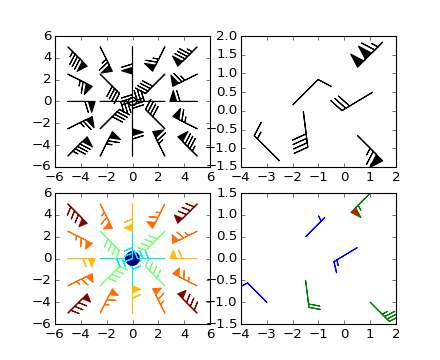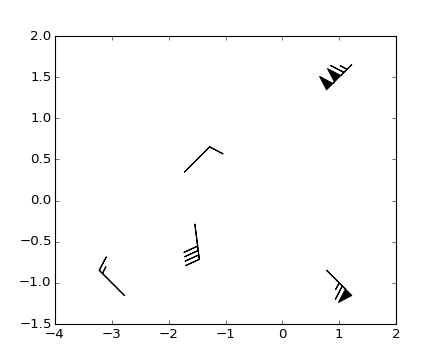#### Previous topic

pylab_examples example code: bar_stacked.py

#### Next topic

pylab_examples example code: barchart_demo.py

# pylab_examples example code: barb_demo.py¶(png, hires.png, pdf)(png, hires.png, pdf)

```'''
Demonstration of wind barb plots
'''
import matplotlib.pyplot as plt
import numpy as np

x = np.linspace(-5, 5, 5)
X,Y = np.meshgrid(x, x)
U, V = 12*X, 12*Y

data = [(-1.5, .5, -6, -6),
(1, -1, -46, 46),
(-3, -1, 11, -11),
(1, 1.5, 80, 80),
(0.5, 0.25, 25, 15),
(-1.5, -0.5, -5, 40)]

data = np.array(data, dtype=[('x', np.float32), ('y', np.float32),
('u', np.float32), ('v', np.float32)])

#Default parameters, uniform grid
ax = plt.subplot(2,2,1)
ax.barbs(X, Y, U, V)

#Arbitrary set of vectors, make them longer and change the pivot point
#(point around which they're rotated) to be the middle
ax = plt.subplot(2,2,2)
ax.barbs(data['x'], data['y'], data['u'], data['v'], length=8, pivot='middle')

#Showing colormapping with uniform grid.  Fill the circle for an empty barb,
#don't round the values, and change some of the size parameters
ax = plt.subplot(2,2,3)
ax.barbs(X, Y, U, V, np.sqrt(U*U + V*V), fill_empty=True, rounding=False,
sizes=dict(emptybarb=0.25, spacing=0.2, height=0.3))

#Change colors as well as the increments for parts of the barbs
ax = plt.subplot(2,2,4)
ax.barbs(data['x'], data['y'], data['u'], data['v'], flagcolor='r',
barbcolor=['b','g'], barb_increments=dict(half=10, full=20, flag=100),
flip_barb=True)